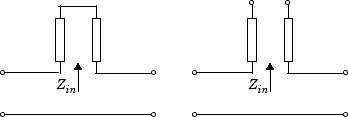Documentation

# RLCG Transmission Line

Model RLCG transmission line

## Library

Transmission Lines sublibrary of the Physical library## Description

The RLCG Transmission Line block models the RLCG transmission line described in the block dialog box in terms of its frequency-dependent resistance, inductance, capacitance, and conductance. The transmission line, which can be lossy or lossless, is treated as a two-port linear network.where z′ = z + Δz.

The block lets you model the transmission line as a stub or as a stubless line.

### Stubless Transmission Line

If you model an RLCG transmission line as a stubless line, the RLCG Transmission Line block first calculates the ABCD-parameters at each frequency contained in the modeling frequencies vector. It then uses the `abcd2s` function to convert the ABCD-parameters to S-parameters.

The block calculates the ABCD-parameters using the physical length of the transmission line, d, and the complex propagation constant, k, using the following equations:

`$\begin{array}{l}A=\frac{{e}^{kd}+{e}^{-kd}}{2}\\ B=\frac{{Z}_{0}*\left({e}^{kd}-{e}^{-kd}\right)}{2}\\ C=\frac{{e}^{kd}-{e}^{-kd}}{2*{Z}_{0}}\\ D=\frac{{e}^{kd}+{e}^{-kd}}{2}\end{array}$`

Z0 and k are vectors whose elements correspond to the elements of f, a vector of modeling frequencies. Both can be expressed in terms of the resistance (R), inductance (L), conductance (G), and capacitance (C) per unit length (meters) as follows:

`$\begin{array}{c}{Z}_{0}=\sqrt{\frac{R+j\omega L}{G+j\omega C}}\\ k={k}_{r}+j{k}_{i}=\sqrt{\left(R+j\omega L\right)\left(G+j\omega C\right)}\end{array}$`

### Shunt and Series Stubs

If you model the transmission line as a shunt or series stub, the RLCG Transmission Line block first calculates the ABCD-parameters at each frequency contained in the vector of modeling frequencies. It then uses the `abcd2s` function to convert the ABCD-parameters to S-parameters.

### Shunt ABCD-Parameters

When you set the Stub mode parameter in the mask dialog box to `Shunt`, the two-port network consists of a stub transmission line that you can terminate with either a short circuit or an open circuit as shown here.Zin is the input impedance of the shunt circuit. The ABCD-parameters for the shunt stub are calculated as

`$\begin{array}{c}A=1\\ B=0\\ C=1/{Z}_{in}\\ D=1\end{array}$`

### Series ABCD-Parameters

When you set the Stub mode parameter in the mask dialog box to `Series`, the two-port network consists of a series transmission line that you can terminate with either a short circuit or an open circuit as shown here.Zin is the input impedance of the series circuit. The ABCD-parameters for the series stub are calculated as

`$\begin{array}{c}A=1\\ B={Z}_{in}\\ C=0\\ D=1\end{array}$`

## Parameters

### Main Tab

Resistance per length (ohms/m)

Vector of resistance values in ohms per meter.

Inductance per length (H/m)

Vector of inductance values in henries per meter.

Capacitance per length (F/m)

Vector of capacitance values in farads per meter.

Conductance per length (S/m)

Vector of conductance values in siemens per meter.

Frequency (Hz)

Vector of frequency values at which the resistance, inductance, capacitance, and conductance values are known.

Interpolation method

Specify the interpolation method the block uses to calculate the parameter values at the modeling frequencies. Your choices are `Linear`, `Spline`, or `Cubic`.

Transmission line length (m)

Physical length of the transmission line.

Stub mode

Type of stub. Your choices are `Not a stub`, `Shunt`, or `Series`.

Termination of stub

Stub termination for stub modes `Shunt` and `Series`. Choices are `Open` or `Short`. This parameter becomes visible only when Stub mode is set to `Shunt` or `Series`.

### Visualization Tab

For information about plotting, see Create Plots.

## References

 Pozar, David M. Microwave Engineering, John Wiley & Sons, Inc., 2005.